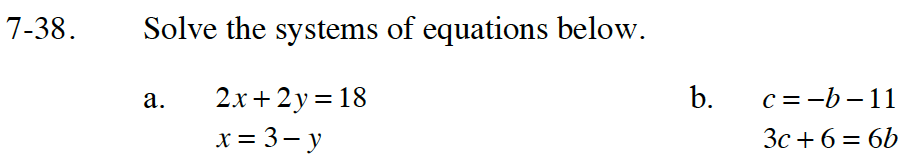Home > CC4 > Chapter 7 > Lesson 7.1.3 > Problem7-38

7-38.
1. Solve the systems of equations below. Homework Help ✎

2.  a. 2x + 2y = 18 x = 3 − y b. c = −b − 11 3c + 6 = 6bRead the Math Notes box in Lesson 6.2.3 to review the Substitution Method. Use it to solve both parts.

Read the Math Notes box in Lesson 6.4.1 to review the different types of solutions for systems of equations.

Substitute (3 − y) in for x, in the equation 2x + 2y = 18, so 2(3 − y) + 2y = 18.

Solve for y.

Substitute the value for y into either of the original equations. Solve for x.

No Solution because when solving for y, the equation shows 6 = 18 and 6 ≠18.